# Презентация, доклад Rotation of rigid bodies. Angular momentum and torque. Properties of fluids

Вы можете изучить и скачать доклад-презентацию на тему Rotation of rigid bodies. Angular momentum and torque. Properties of fluids. Презентация на заданную тему содержит 37 слайдов. Для просмотра воспользуйтесь проигрывателем, если материал оказался полезным для Вас - поделитесь им с друзьями с помощью социальных кнопок и добавьте наш сайт презентаций в закладки!
Презентации» Физика» Rotation of rigid bodies. Angular momentum and torque. Properties of fluids

Слайды и текст этой презентации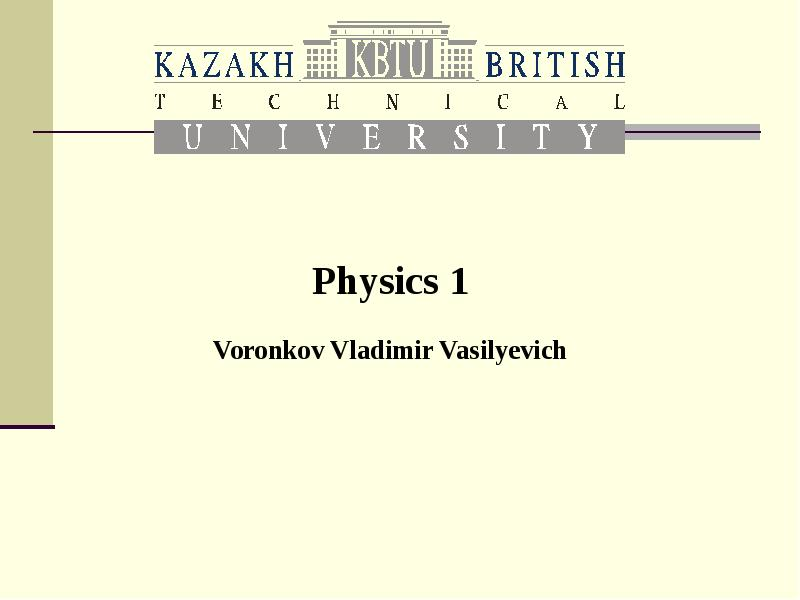Описание слайда: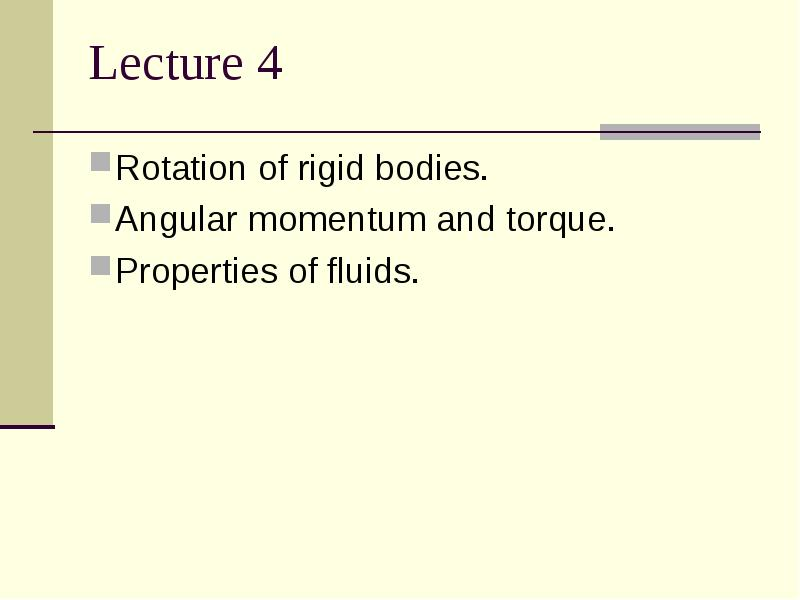Описание слайда:
Lecture 4 Rotation of rigid bodies. Angular momentum and torque. Properties of fluids.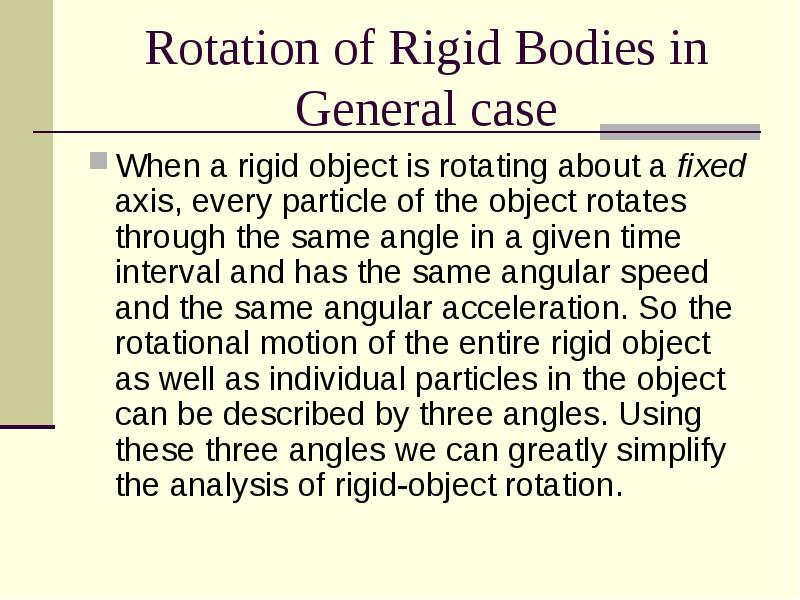Описание слайда:
Rotation of Rigid Bodies in General case When a rigid object is rotating about a fixed axis, every particle of the object rotates through the same angle in a given time interval and has the same angular speed and the same angular acceleration. So the rotational motion of the entire rigid object as well as individual particles in the object can be described by three angles. Using these three angles we can greatly simplify the analysis of rigid-object rotation.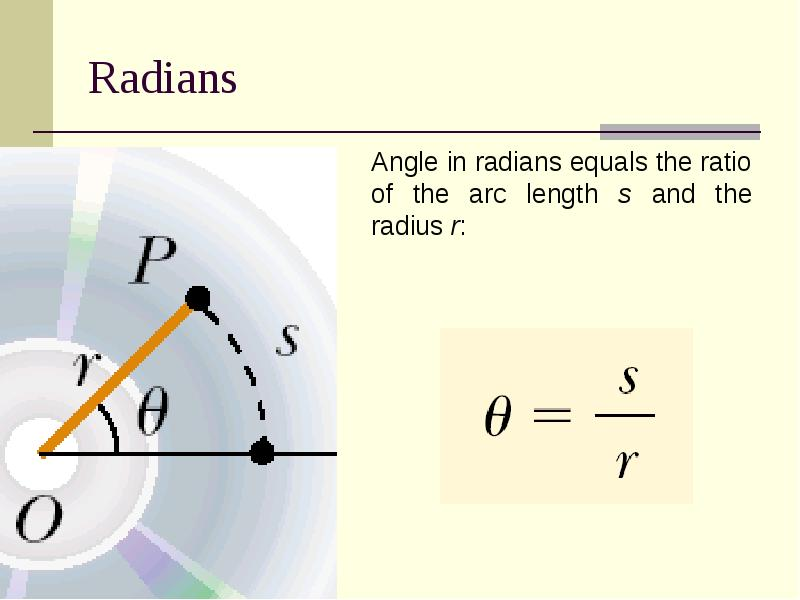Описание слайда: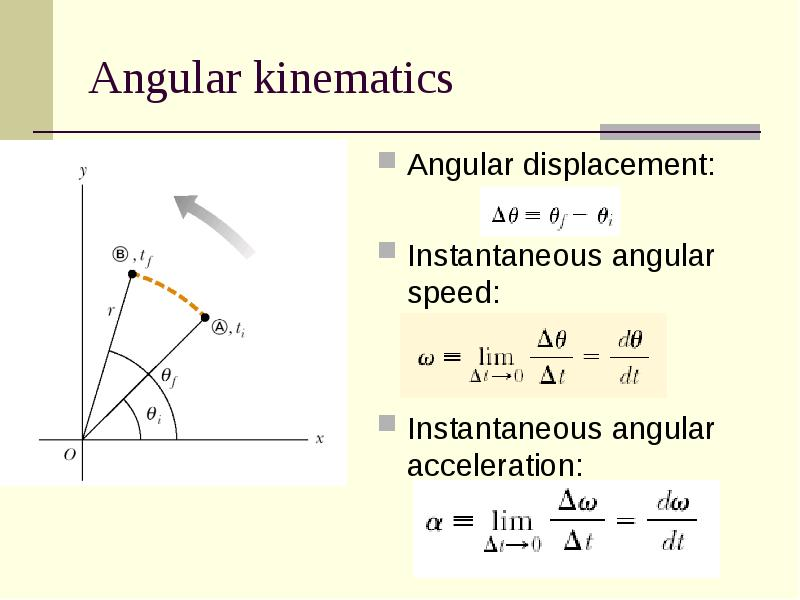Описание слайда:
Angular kinematics Angular displacement: Instantaneous angular speed: Instantaneous angular acceleration: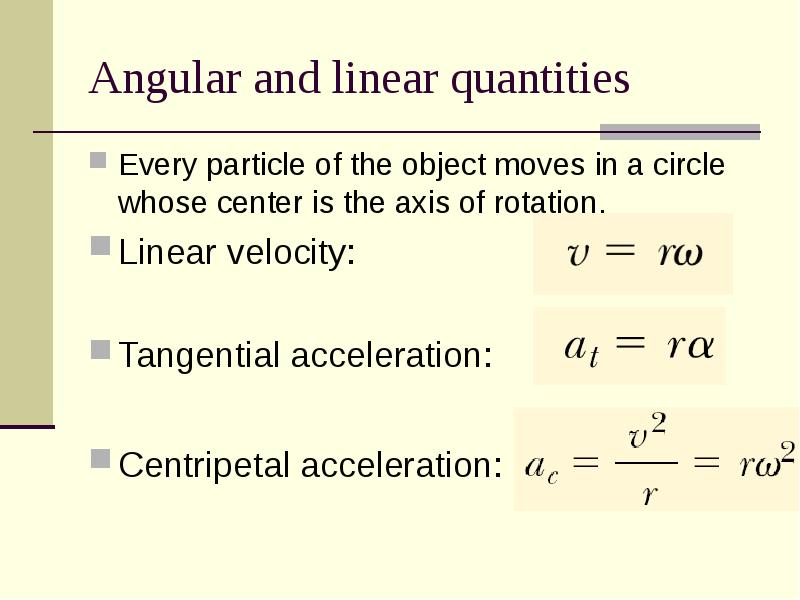Описание слайда:
Angular and linear quantities Every particle of the object moves in a circle whose center is the axis of rotation. Linear velocity: Tangential acceleration: Centripetal acceleration: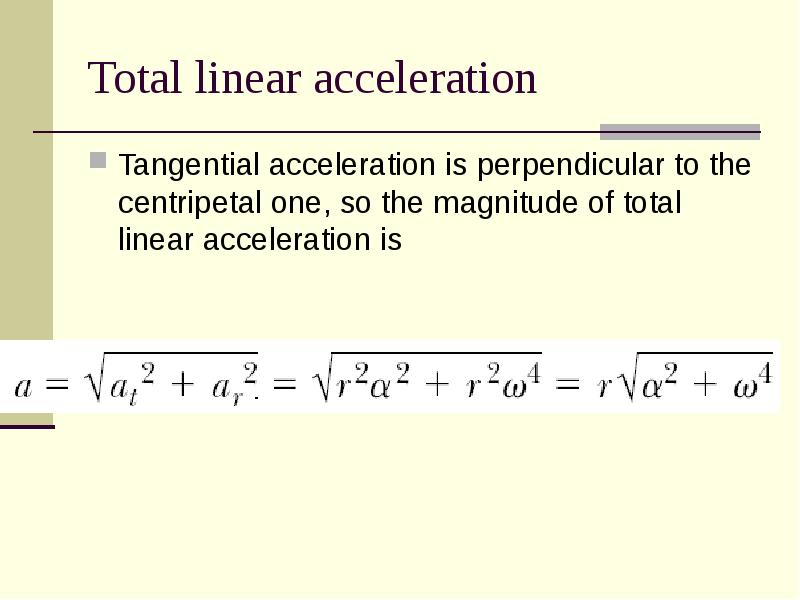Описание слайда:
Total linear acceleration Tangential acceleration is perpendicular to the centripetal one, so the magnitude of total linear acceleration isОписание слайда:
Angular velocity Angular velocity is a vector.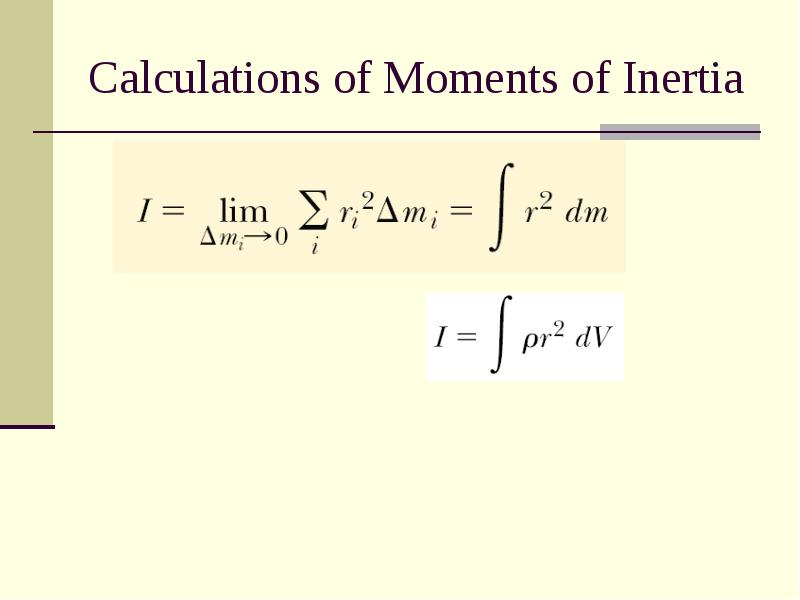Описание слайда:
Calculations of Moments of Inertia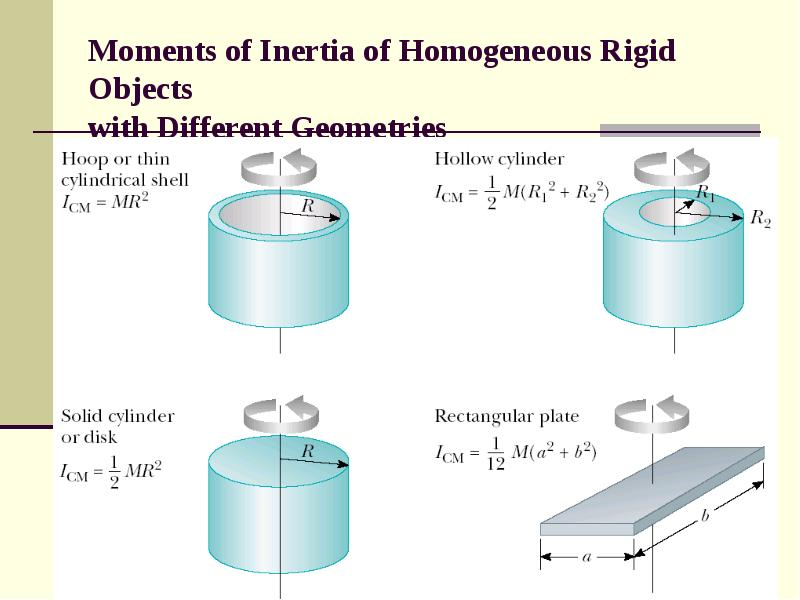Описание слайда:
Moments of Inertia of Homogeneous Rigid Objects with Different Geometries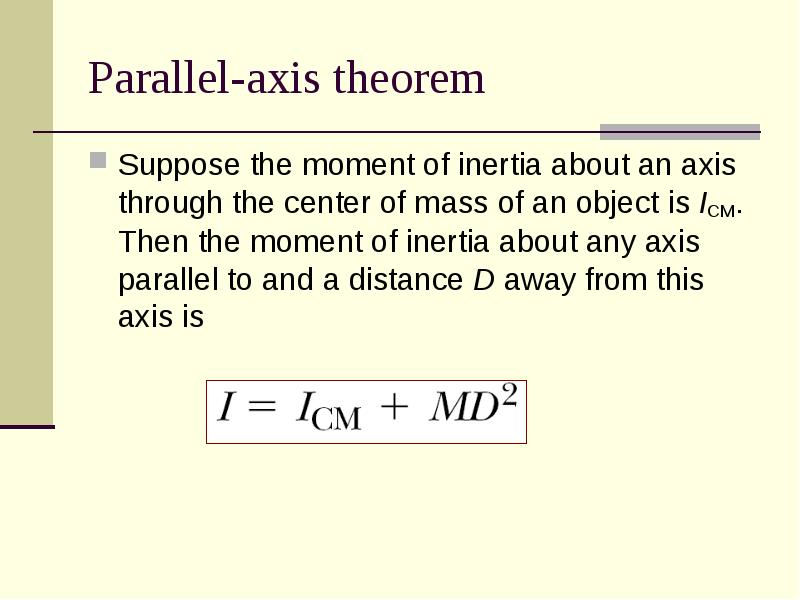Описание слайда:
Parallel-axis theorem Suppose the moment of inertia about an axis through the center of mass of an object is ICM. Then the moment of inertia about any axis parallel to and a distance D away from this axis is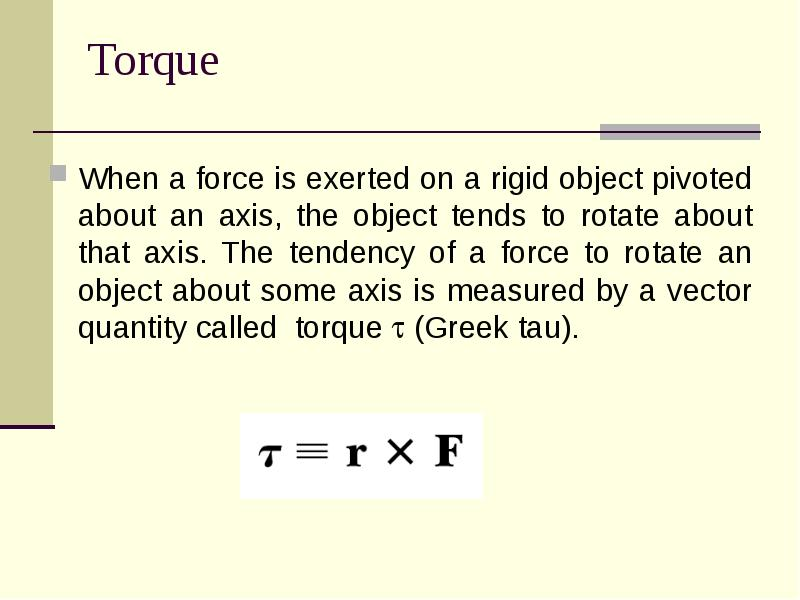Описание слайда:
Torque When a force is exerted on a rigid object pivoted about an axis, the object tends to rotate about that axis. The tendency of a force to rotate an object about some axis is measured by a vector quantity called torque  (Greek tau).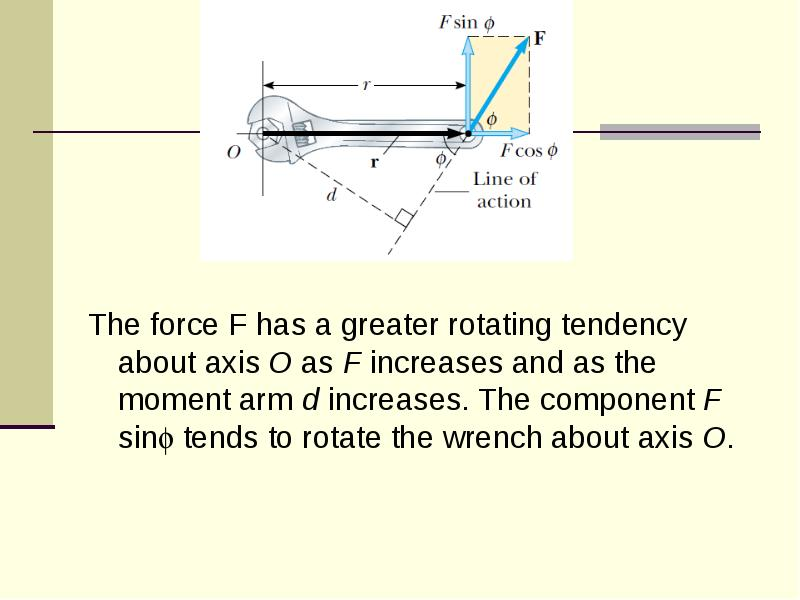Описание слайда:
The force F has a greater rotating tendency about axis O as F increases and as the moment arm d increases. The component F sin tends to rotate the wrench about axis O.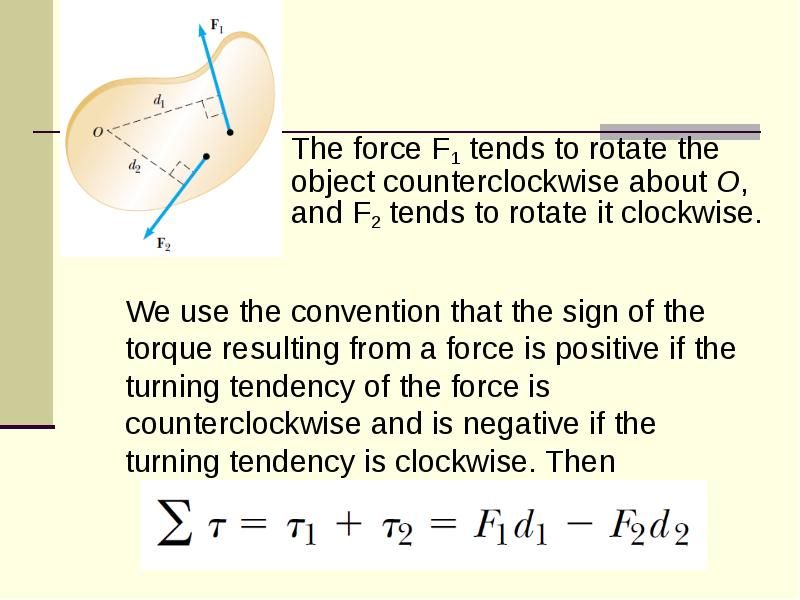Описание слайда:
We use the convention that the sign of the torque resulting from a force is positive if the turning tendency of the force is counterclockwise and is negative if the turning tendency is clockwise. Then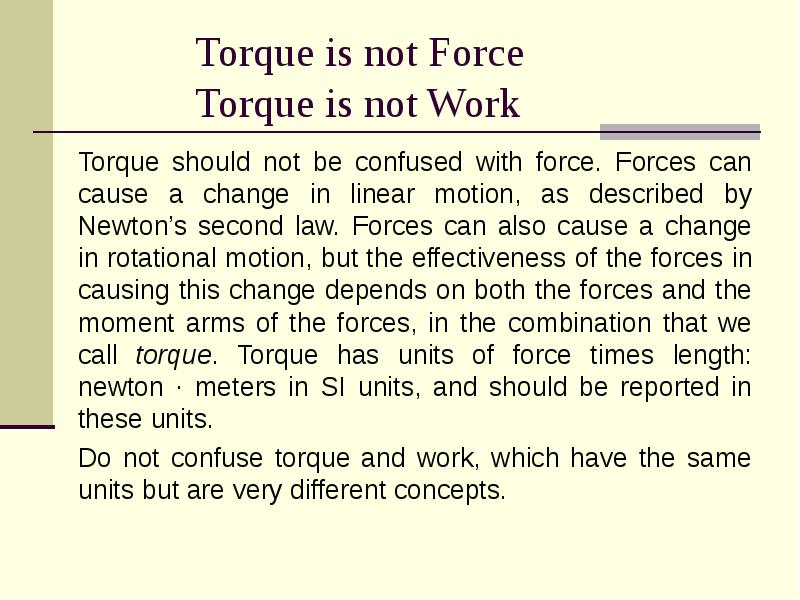Описание слайда:
Torque is not Force Torque is not Work Torque should not be confused with force. Forces can cause a change in linear motion, as described by Newton’s second law. Forces can also cause a change in rotational motion, but the effectiveness of the forces in causing this change depends on both the forces and the moment arms of the forces, in the combination that we call torque. Torque has units of force times length: newton · meters in SI units, and should be reported in these units. Do not confuse torque and work, which have the same units but are very different concepts.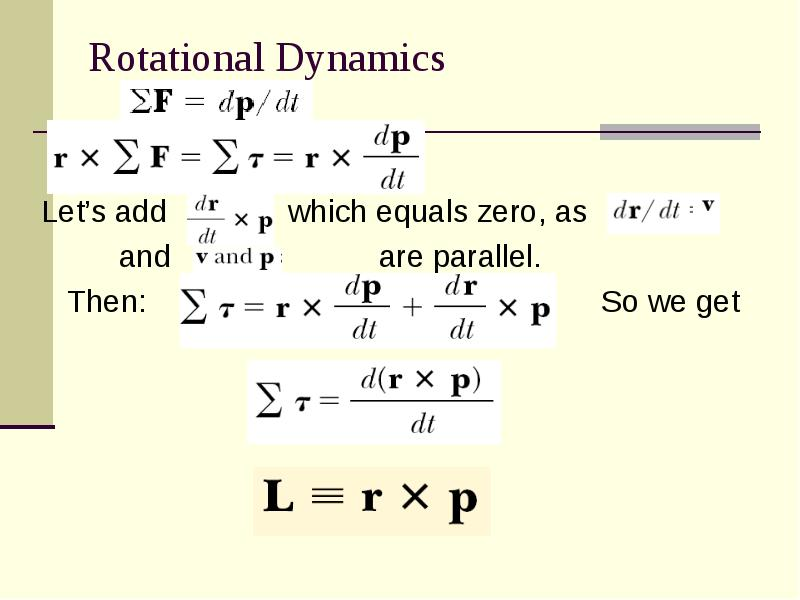Описание слайда:
Rotational Dynamics Let’s add which equals zero, as and are parallel. Then: So we get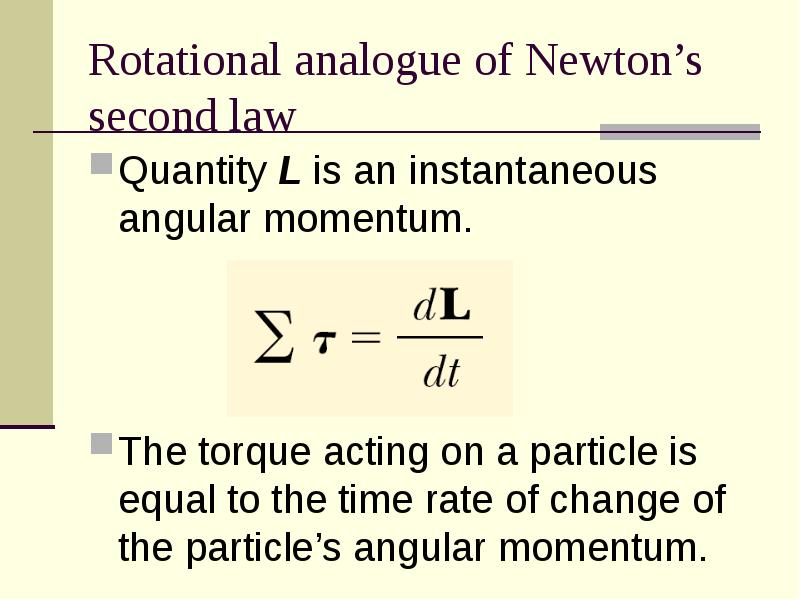Описание слайда:
Rotational analogue of Newton’s second law Quantity L is an instantaneous angular momentum. The torque acting on a particle is equal to the time rate of change of the particle’s angular momentum.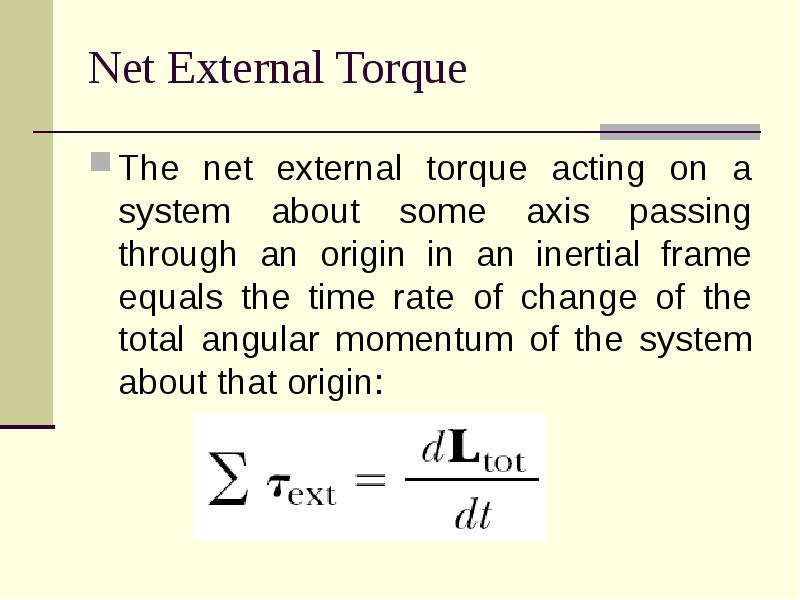Описание слайда:
Net External Torque The net external torque acting on a system about some axis passing through an origin in an inertial frame equals the time rate of change of the total angular momentum of the system about that origin: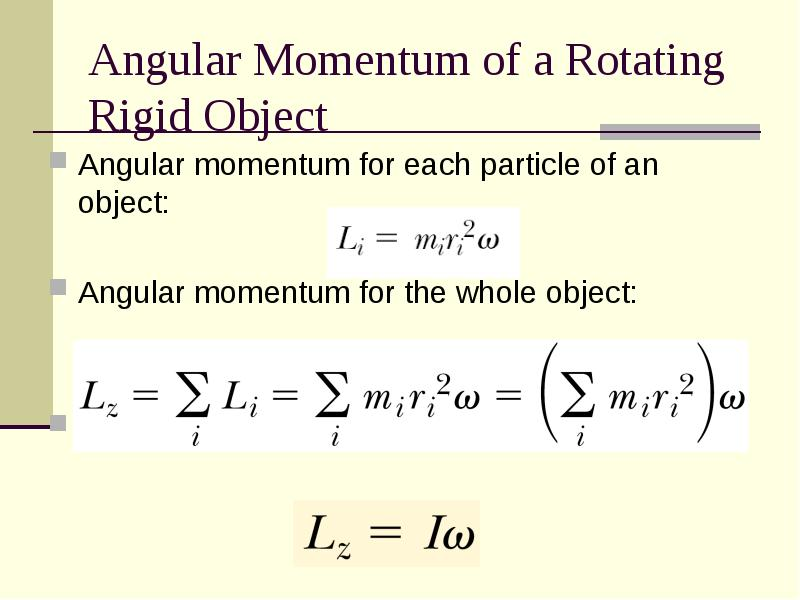Описание слайда:
Angular Momentum of a Rotating Rigid Object Angular momentum for each particle of an object: Angular momentum for the whole object: Thus: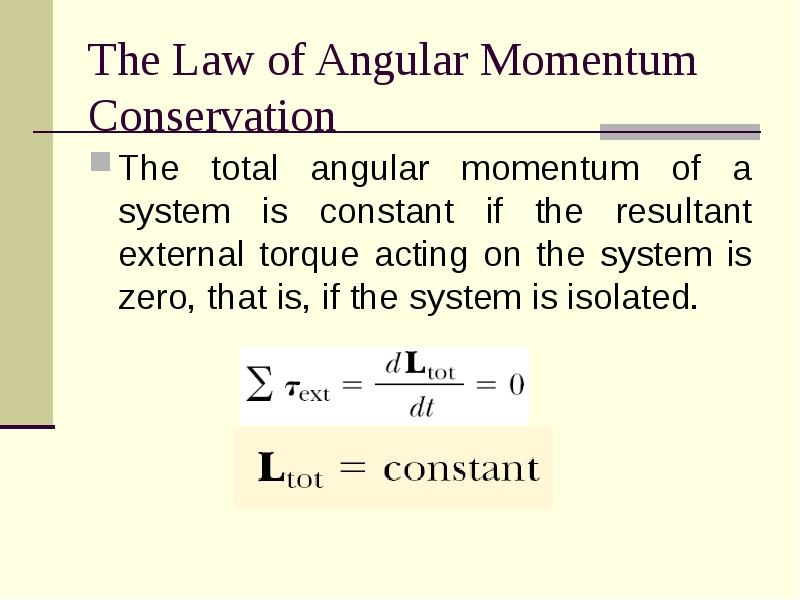Описание слайда:
The Law of Angular Momentum Conservation The total angular momentum of a system is constant if the resultant external torque acting on the system is zero, that is, if the system is isolated.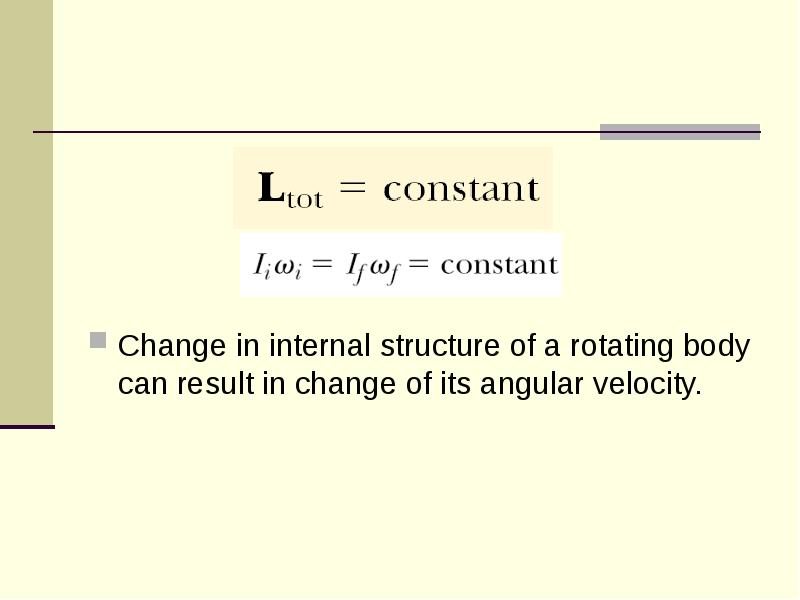Описание слайда:
Change in internal structure of a rotating body can result in change of its angular velocity.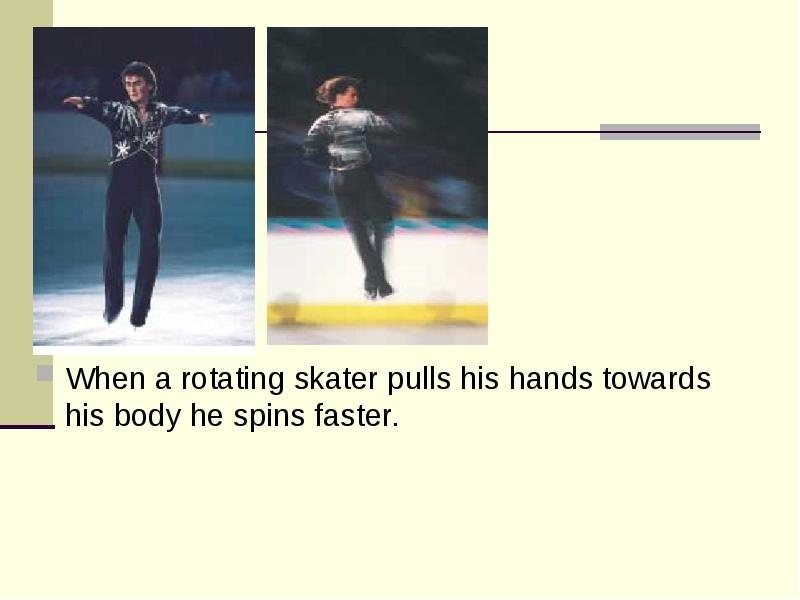Описание слайда:
When a rotating skater pulls his hands towards his body he spins faster.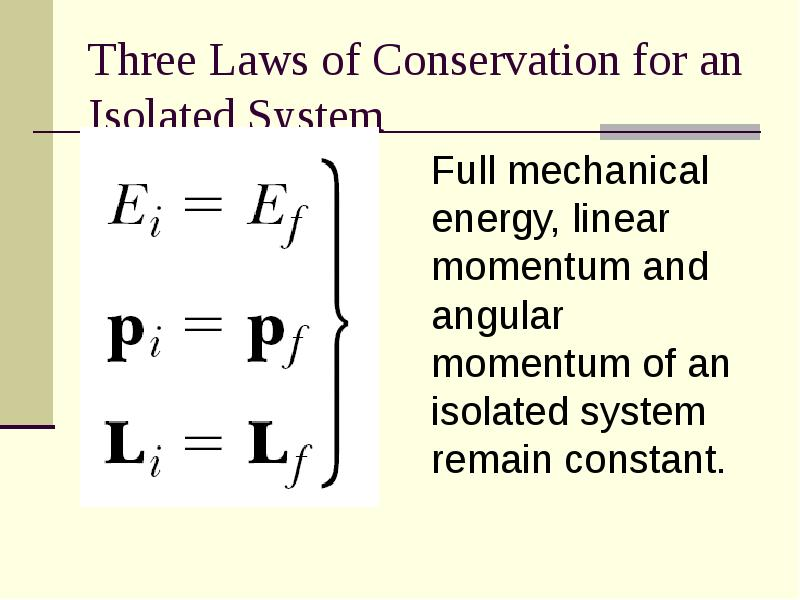Описание слайда:
Three Laws of Conservation for an Isolated System Full mechanical energy, linear momentum and angular momentum of an isolated system remain constant.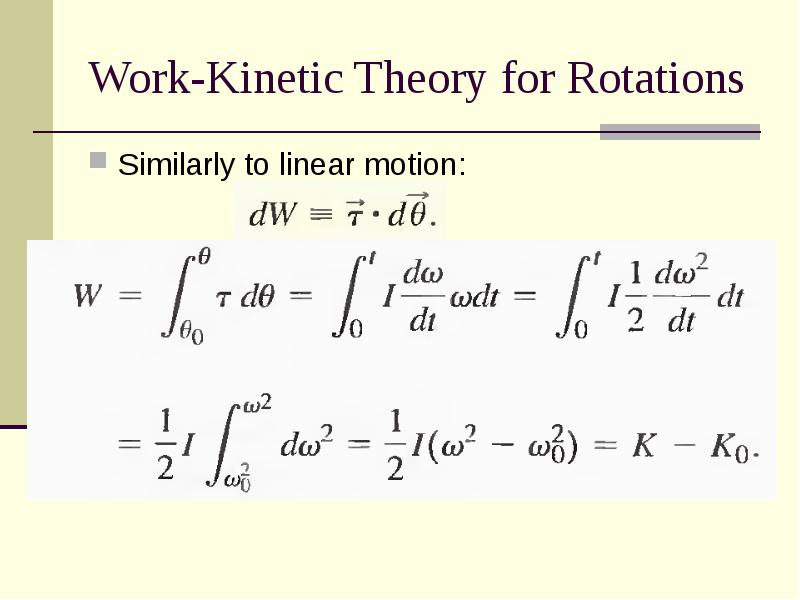Описание слайда:
Work-Kinetic Theory for Rotations Similarly to linear motion: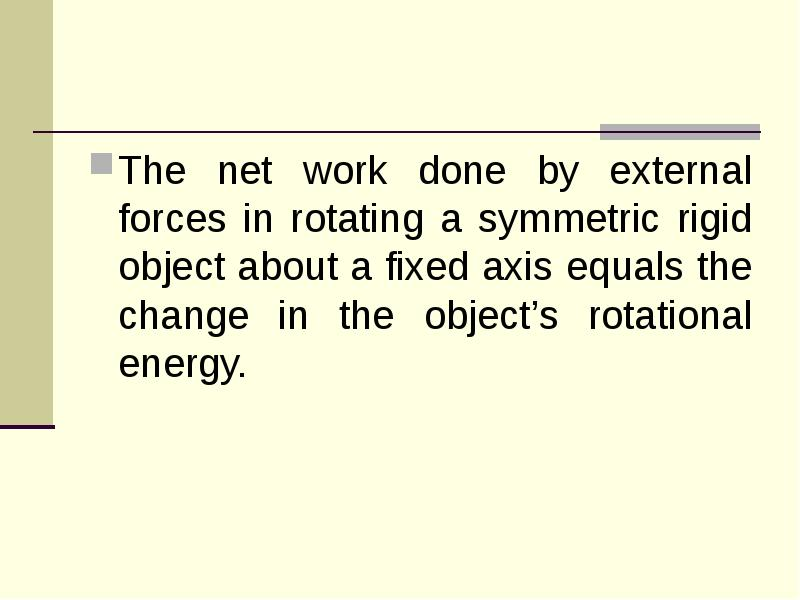Описание слайда:
The net work done by external forces in rotating a symmetric rigid object about a fixed axis equals the change in the object’s rotational energy.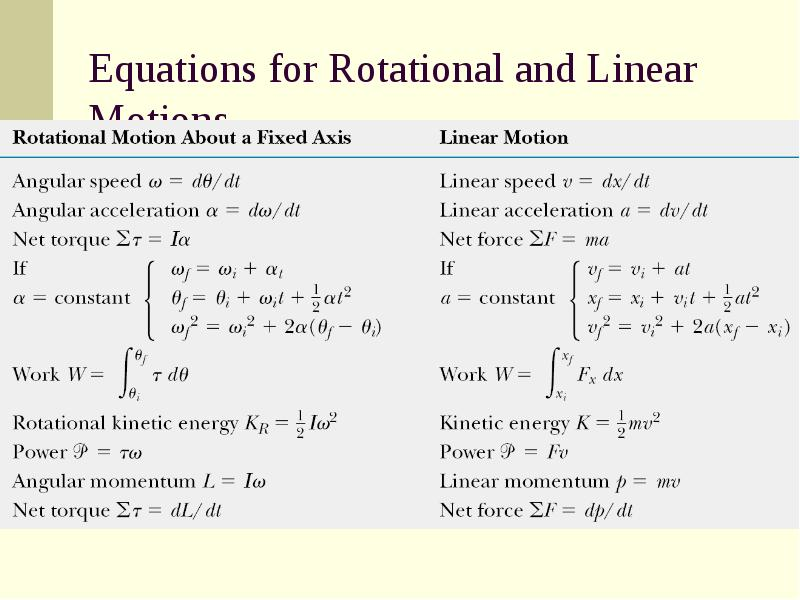Описание слайда:
Equations for Rotational and Linear Motions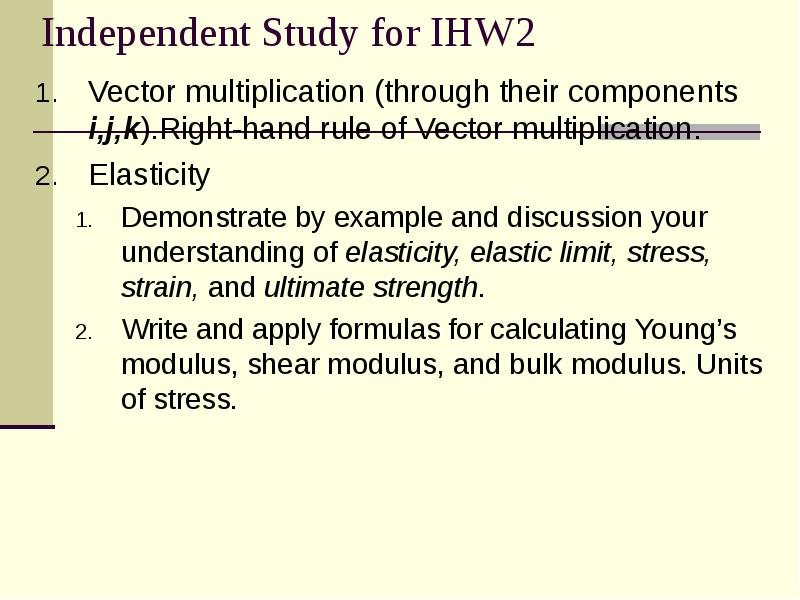Описание слайда:
Independent Study for IHW2 Vector multiplication (through their components i,j,k).Right-hand rule of Vector multiplication. Elasticity Demonstrate by example and discussion your understanding of elasticity, elastic limit, stress, strain, and ultimate strength. Write and apply formulas for calculating Young’s modulus, shear modulus, and bulk modulus. Units of stress.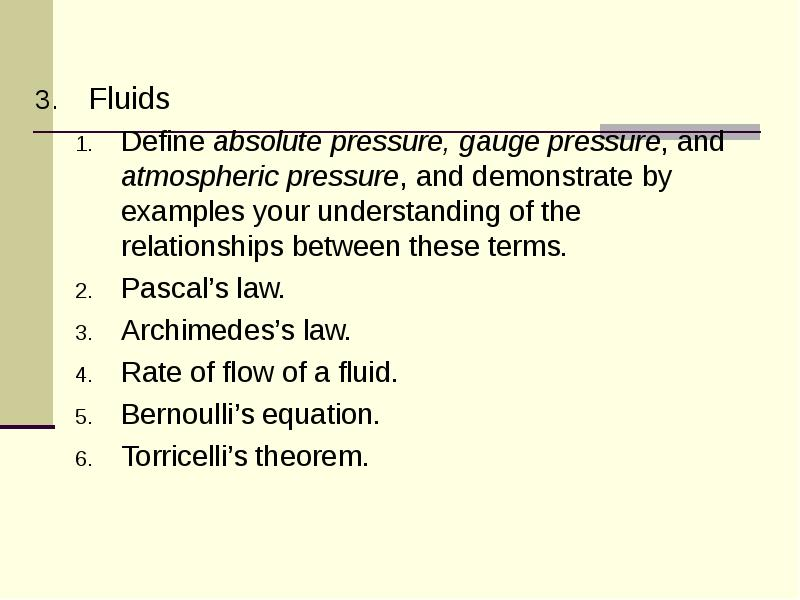Описание слайда:
Fluids Fluids Define absolute pressure, gauge pressure, and atmospheric pressure, and demonstrate by examples your understanding of the relationships between these terms. Pascal’s law. Archimedes’s law. Rate of flow of a fluid. Bernoulli’s equation. Torricelli’s theorem.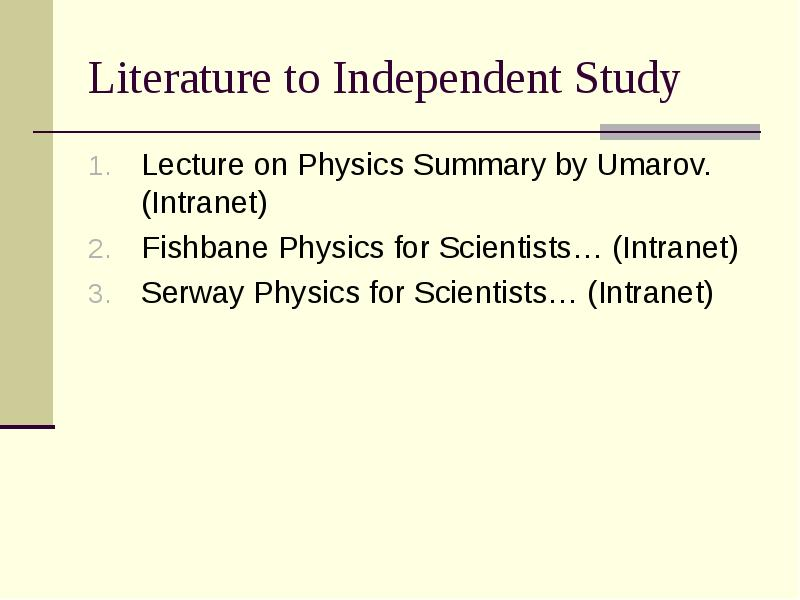Описание слайда:
Literature to Independent Study Lecture on Physics Summary by Umarov. (Intranet) Fishbane Physics for Scientists… (Intranet) Serway Physics for Scientists… (Intranet)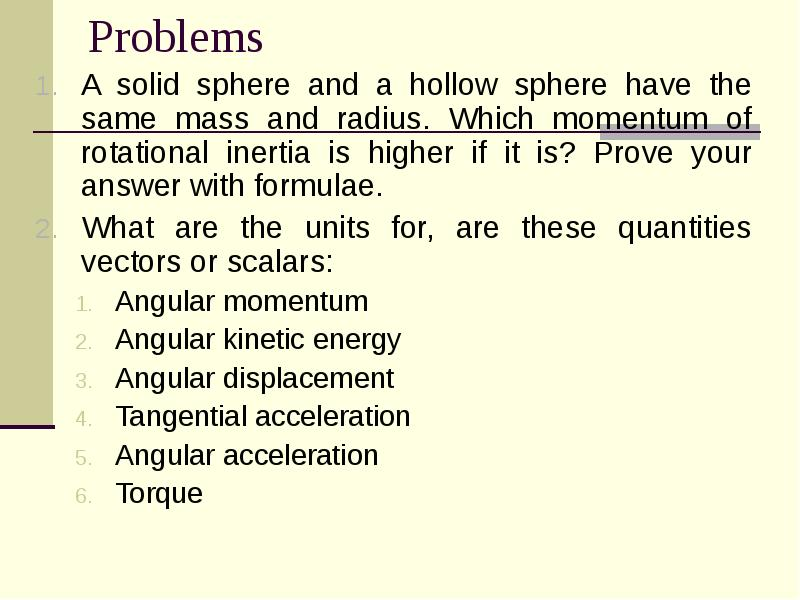Описание слайда:
Problems A solid sphere and a hollow sphere have the same mass and radius. Which momentum of rotational inertia is higher if it is? Prove your answer with formulae. What are the units for, are these quantities vectors or scalars: Angular momentum Angular kinetic energy Angular displacement Tangential acceleration Angular acceleration Torque

Скачать презентацию на тему Rotation of rigid bodies. Angular momentum and torque. Properties of fluids можно ниже:

Похожие презентации